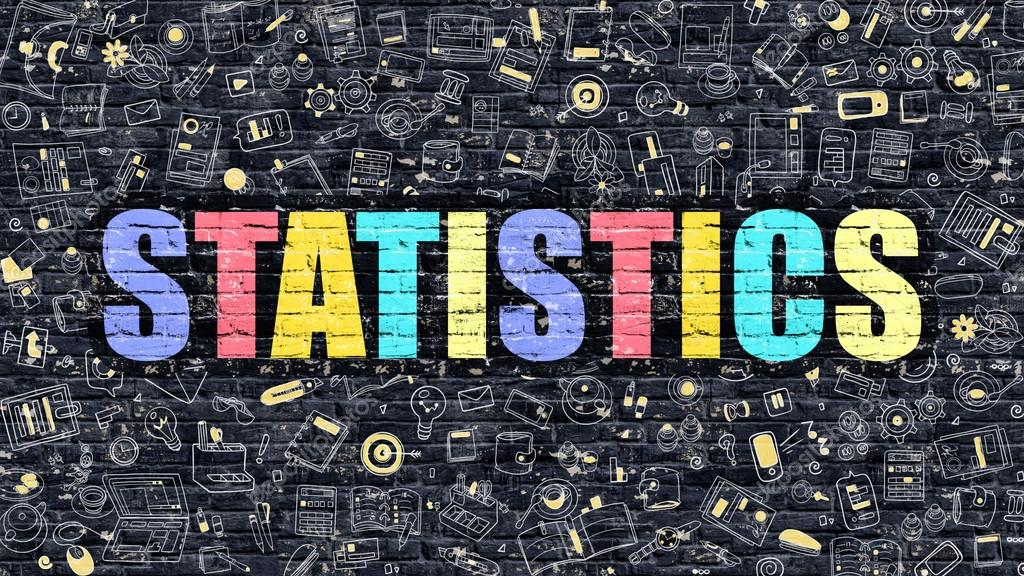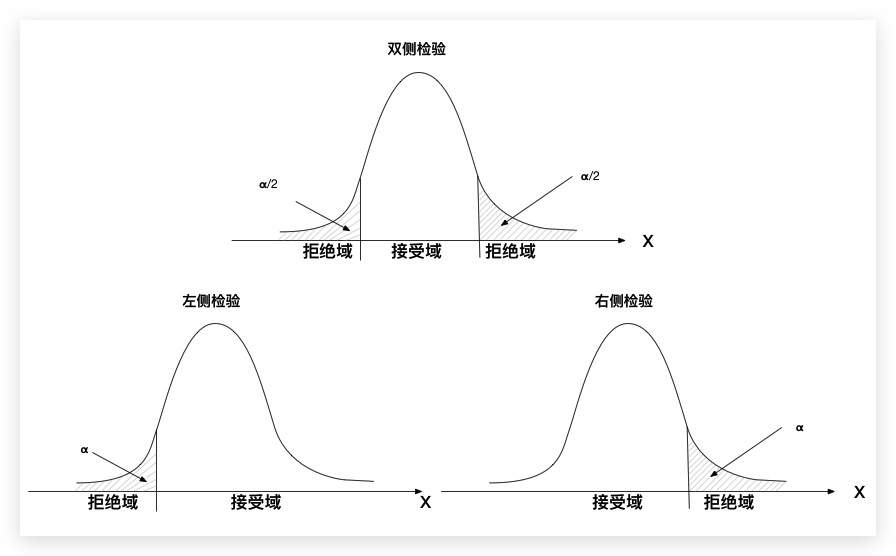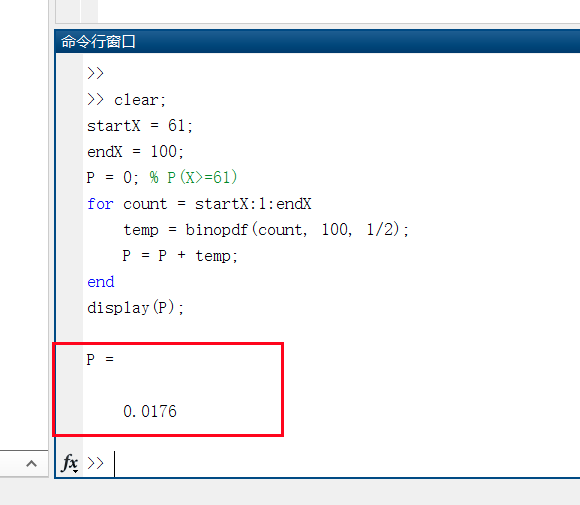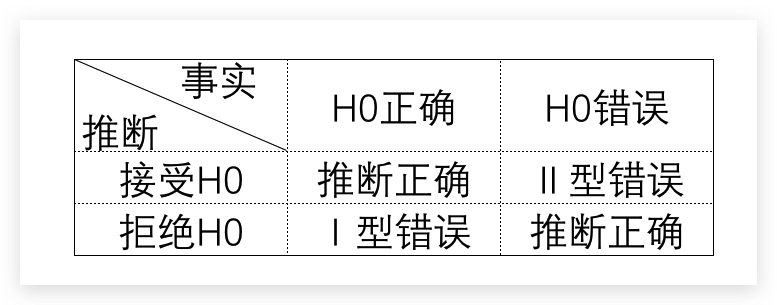# xyZGHio

0%# 基本原理

• 当 $p \leq \alpha$ 时，说明在$H_0$假设条件下，出现给定的样本数据是一个小概率事件，而实际上样本数据确实如此，即发生了不合理现象。故认为$H_0$假设是错误的、被证伪。从而，拒绝$H_0$假设，接受$H_1$假设
• 当 $p > \alpha$ 时，说明在$H_0$假设条件下，出现给定的样本数据不是一个小概率事件，出现给定的样本数据是合理的，故$H_0$假设被接受，而$H_1$假设被拒绝# 小试牛刀

$H_1$: 甲获胜的概率 > 1/2

$H_0$: 甲获胜的概率 = 1/2# 假设检验的两类错误

• Ⅰ型错误: 当虚无假设$H_0$实际上是正确的，我们却错误地拒绝了它。又叫作第一类错误、$\alpha$错误
• Ⅱ型错误: 当虚无假设$H_0$实际上是错误的，我们却错误地接受了它。又叫作第二类错误、$\beta$错误